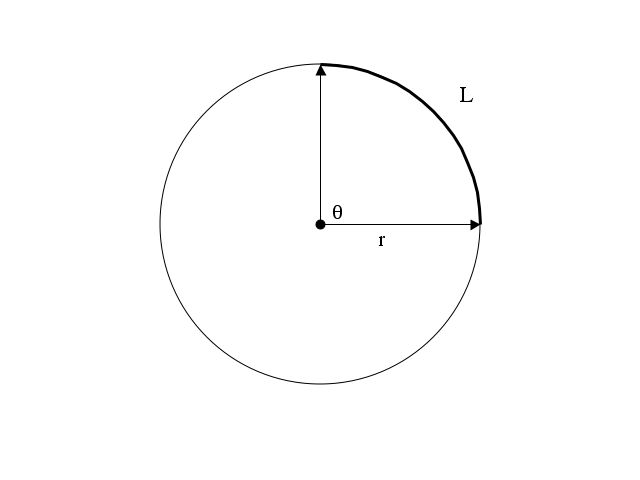Most of the time we measure angles in degrees. For example, there are 360° in a full circle or one cycle of a sine wave, and sin(30°) = 0.5 and cos(90°) = 0. But it turns out that a more natural measure for angles, at least in mathematics, is in radians.

An angle measured in radians is the ratio of the arc length of a circle subtended by that angle, divided by the radius of the circle. To put that mouth full of words into a diagram, the figure below shows an angle of 90°, the arc length L subtended by that angle, and the radius r of the circle.The circumference of the entire circle is (2 π r); the arc length of the 1/4 of that circle subtended by this angle is L = (2 π r) / 4 = (π r) / 2; and the ratio of that arc length L to the radius r is π / 2. So 90° = π / 2 radians.

We usually suppress the unit of measurement "radians" since it is understood if no other units for angles is specified. Also, since an angle in radians is defined as the ratio of two lengths, L/r, it is dimensionless.

Since 90° = π / 2 radians, to four significant figures, one radian equals 180°/ π = 57.30°.

There are 2π radians in a full circle. (So 2π radians should equal 360°. Check it out by multiplying 57.30° by 2π = 6.283. You should get 360° to four significant figures.)

30°, which is 1/12 of a full circle, therefore equals 2π/12 = π/6 radians and sin(π/6) = 0.5

90°, which is 1/4 of a full circle, therefore equals 2π/4 =π/2 radians, and cos(π/2) = 0

So when I wrote

s(t) = sin(2 π 3000 t)

to express one of the signals in our discussion with angles measured in radians, I could instead have written

s(t) = sin(1,080,000° t)

which is the equivalent expression when angles are measured in degrees. That's because there are 360° in a cycle (a full circle) so 3000 cycles per second equals

3000 cycles/sec x 360°/cycle = 1,080,000°/sec.

Why are radians more natural than degrees? Comparing the first and second above expressions for s(t), it is easier to see that the frequency is 3 kHz (3000 cycles per second) when angles are measured in radians – provided I leave the 2π factor as an explicit multiplier, which mathematicians and engineers always do.

A second, more fundamental reason that radians are more natural can be seen from the "power series" for sine and cosine. These are infinite series that converge to the sine and cosine functions. If angles are measured in radians then

sin(x) = x - x3/3! + x5/5! - x7/7! + x9/9! ...

and

cos(x) = 1 - x2/2! + x4/4! - x6/6! + x8/8! ...

The same power series would be less "elegant" (a favorite word of mathematicians) if angles are measured in degrees:

sin(x) = (π x / 180) - (π x / 180)3 / 3! + (π x / 180)5 / 5! - (π x / 180) x7 / 7! + π x9 / 9! ...

and

cos(x) = 1 - (π x / 180)2 / 2! + (π x / 180)4 / 4! - (π x / 180)6/ 6! + (π x / 180)8 / 8! ...

As an exercise, you may want to take the power series for sin(x) where x is in radians, substitute x = π/6 = 0.5236 and see how close it comes to 0.5, the correct value for sin(30°) as you vary the number of terms. [Hint: It takes so few terms that you can do it easily on a non-programmable pocket calculator.]

If you liked that first exercise, you may want to try the power series for cos(x) with x = π/2 = 1.5708 and see how fast it converges to 0. While this computation, too, can be done on a non-programmable pocket calculator, it requires a few more terms and you might want to use a spreadsheet program to help.